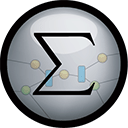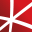# Top 20 NuGet matrix Packages

Math.NET Numerics is the numerical foundation of the Math.NET project, aiming to provide methods and algorithms for numerical computations in science, engineering and every day use. Supports .Net Framework 4.0 or higher and .Net Standard 1.3 or higher, on Windows, Linux and Mac.## Math.NET Numerics - Strong Name Edition

Math.NET Numerics is the numerical foundation of the Math.NET project, aiming to provide methods and algorithms for numerical computations in science, engineering and every day use. Supports .Net Framework 4.0 or higher and .Net Standard 1.3 or higher, on Windows, Linux and Mac. This package contain...
F# Modules for Math.NET Numerics, the numerical foundation of the Math.NET project, aiming to provide methods and algorithms for numerical computations in science, engineering and every day use. Supports .Net Framework 4.5 or higher and .Net Standard 1.6 or higher, on Windows, Linux and Mac.
Google Places, Maps, Roads, Search and Translate. Requests and Responses are complete with Google api documentation and references.## Math.NET Numerics for F# - Strong Name Edition

F# Modules for Math.NET Numerics, the numerical foundation of the Math.NET project, aiming to provide methods and algorithms for numerical computations in science, engineering and every day use. Supports .Net Framework 4.5 or higher and .Net Standard 1.6 or higher, on Windows, Linux and Mac. This pa...
A library for advanced numerical computing, with support for data wrangling, statistics, matrix algebra, advanced functions, numerical integration and optimization, and Fourier transforms.
ZXing.Net is a port of ZXing, an open-source, multi-format 1D/2D barcode image processing library originally implemented in Java. It has been ported by hand with a lot of optimizations and improvements. It is compatible with .Net 2.0, .Net 3.5, .Net 4.0, .Net 4.5, .Net 4.6, .Net 4.7, .Net 4.8, Wind...
Foundational classes for financial, engineering, and scientific applications, including complex number classes, general vector and matrix classes, structured sparse matrix classes and factorizations, general sparse matrix classes and factorizations, general matrix decompositions, least squares solut...
The GPU-accelerated version of package CenterSpace.NMath. With a few minor exceptions, such as optional GPU configuration settings, the API is identical between CenterSpace.NMath.Premium and CenterSpace.NMath. If using at least .NET Framework 4.6.1 or .NET Core 2.0, we recommend using one of our NMa...## NMath - Standard Library - Windows x64

Foundational classes for financial, engineering, and scientific applications, including complex number classes, general vector and matrix classes, structured sparse matrix classes and factorizations, general sparse matrix classes and factorizations, general matrix decompositions, least squares solut...
Foundational classes for financial, engineering, and scientific applications, including complex number classes, general vector and matrix classes, structured sparse matrix classes and factorizations, general sparse matrix classes and factorizations, general matrix decompositions, least squares solut...
Avalonia matrix extensions.
This library contains geometric primitives and algorithms: 2D, 3D and 4V vector, matrix, polyline and polygon types. The main geometric operations are 2D (Delaunay) triangulation and polygon clipping (union, difference, intersection, xor). The latest clipping implementation is floating point based a...
Neodynamic ThermalLabel SDK for .NET is a .NET Standard library (DLL) that lets you to design barcode labels and print them to Zebra (ZPL or EPL), EPSON (ESC/POS) &amp; Honeywell-Intermec Fingerprint printers by writing just pure .NET code in VB or C#. ThermalLabel SDK can be used for designing and ...
The Extreme Optimization Numerical Libraries for .NET are a set of libraries for numerical computing and data analysis. This is the main package that contains all the core functionality. For optimal performance, we strongly recommend also referencing one of the native packages based on Intel's Mat...
Contains a matrix extension library, along with a suite of numerical matrix decomposition methods, numerical optimization algorithms for constrained and unconstrained problems, special functions and other tools for scientific applications. This package is part of the Accord.NET Framework.
GlmNet is a .NET version of the popular GLM mathematics library.